Courses

# Beam on Elastic Foundation Civil Engineering (CE) Notes | EduRev

## Civil Engineering (CE) : Beam on Elastic Foundation Civil Engineering (CE) Notes | EduRev

The document Beam on Elastic Foundation Civil Engineering (CE) Notes | EduRev is a part of the Civil Engineering (CE) Course Advanced Solid Mechanics - Notes, Videos, MCQs & PPTs.
All you need of Civil Engineering (CE) at this link: Civil Engineering (CE)

Beam on Elastic Foundation

General formulation

In this section, we formulate the boundary value problem of beam on an elastic foundation. A beam having some cross section, resting on an elastic support is shown in figure 11.1. We assume that the reaction offered by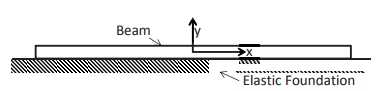Figure 11.1: Schematic of a long beam on elastic foundation

the support at any point is directly proportional to the displacement of that point along the y direction and is in a direction opposite to the displacement. Thus, if ∆ is the vertical displacement of a point in the beam, qy the support reaction per unit width of the beam, then the above assumption that the reaction force is proportional to the displacement mathematically translates into requiring

qy = −Ks∆.                 (11.1)

Assuming the beam to be homogeneous, we obtained the equation (8.41)
which we document here again: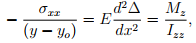(11.2)

where yo is the y coordinate of the centroid of the cross section which can be taken as 0 without loss of generality provided the origin of the coordinate system used is located at the centroid of the cross section, E is the Young’s modulus, (x, y) is the coordinate of the point along the axis of the beam direction and the y direction, Mis the z component of the bending moment, Izz is the moment of inertia of the section about the z axis. In section 8.1, we integrated the equilibrium equations and obtained equations (8.18) and (8.25) which we record here: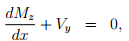(11.3)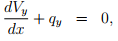(11.4)

where Vis the shear force along the y direction and qy is the transverse loading along the y direction. Combining the equations (11.3) and (11.4) we obtain,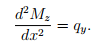(11.5)

Substituting equation (11.2) in equation (11.5), we obtain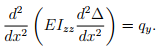(11.6)

Assuming the beam to be homogeneous and prismatic, so that EIzz is constant through the length of the beam, and substituting equation (11.1) in equation (11.6), we obtain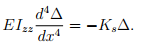(11.7)

Defining,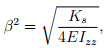(11.8)

equation (11.7) can be written as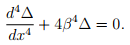(11.9)

The differential equation (11.9) has a general solution:

∆ = exp(−βx)[C1 sin(βx) + Ccos(βx)] + exp(βx)[C3 sin(βx) + C4 cos(βx)],   (11.10)

where Ci ’s are constant to be determined from the boundary conditions. Having found the deflection, the stress is estimated from (11.2) as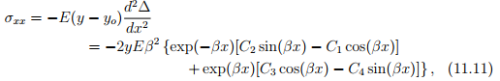where we have assumed that the origin is located at the centroid of the cross section and hence have set yo = 0.

Offer running on EduRev: Apply code STAYHOME200 to get INR 200 off on our premium plan EduRev Infinity!

,

,

,

,

,

,

,

,

,

,

,

,

,

,

,

,

,

,

,

,

,

;